# QANet 模型

## Introduction

在过去几年中最成功的模型通常采用了两项关键要技术：(1) 处理顺序输入的循环模型； (2) 应对长期交互的注意力要素。Seo 等人于 2016 年提出的的双向注意力流 (Bidirectional Attention Flow，BiDAF) 模型将这两项要素成功地结合在了一起，该模型在 SQuAD 数据集上取得了显著的效果。但这类模型的循环特性使得其在训练和推理方面的效率较低，尤其对于长文本而言。昂贵的训练不仅会导致实验的周期延长，限制研究人员进行快速迭代，还会使模型难以使用更大的数据集。此外，缓慢的推理速度也阻碍了机器理解系统在实时应用中的部署。

在本文中，为了使机器理解更高效，提出去掉这些模型的循环性质，仅将卷积和自注意力作为编码器的构成模块，分别对问题和语境进行编码。然后通过标准注意力（standard attentions, Xiong 等人于 2016 年提出；Seo 等人于 2016 年提出；Bahdanau 等人于 2015 年提出）来学习语境和问题之间的交互。在最终对每个作为答案范围的开始或结束位置的概率进行解码之前，用无循环编码器再次对结果表征进行编码。该架构称为 QANet。

模型设计的主要思想：卷积捕获文本的局部结构，而自注意力则学习每对单词之间的全局交互。附加语境疑问注意力 (the additional context-query attention) 是一个标准模块，用于为语境段落中的每个位置构造查询感知的语境向量 (query-aware context vector)，这在随后的建模层中得到使用。

架构的前馈特性极大地提高了模型的效率。在基于 SQuAD 数据集的实验中，模型的训练速度提高了 3 至 13 倍，推理速度提高了 4 至 9 倍。做一个简单的比较，模型可以在 3 个小时的训练中达到与 BiDAF 模型（ Seo 等人于 2016 年提出）相同的精确度（F1 值为 77.0），在此之前，这一过程需花费 15 个小时的时间。加速增益还允许使用更多的迭代来训练模型，以获得比竞争模型更好的结果。例如，模型进行 18 个小时的训练后在开发集上的 F1 值达到了 82.7，这比 Seo 等人于 2016 年提出的模型表现要好很多，可以与已发布过的最佳结果相媲美。

## Model

• input embedding layer
• embedding encoder layer
• context-query attention layer
• model encoder layer
• output layer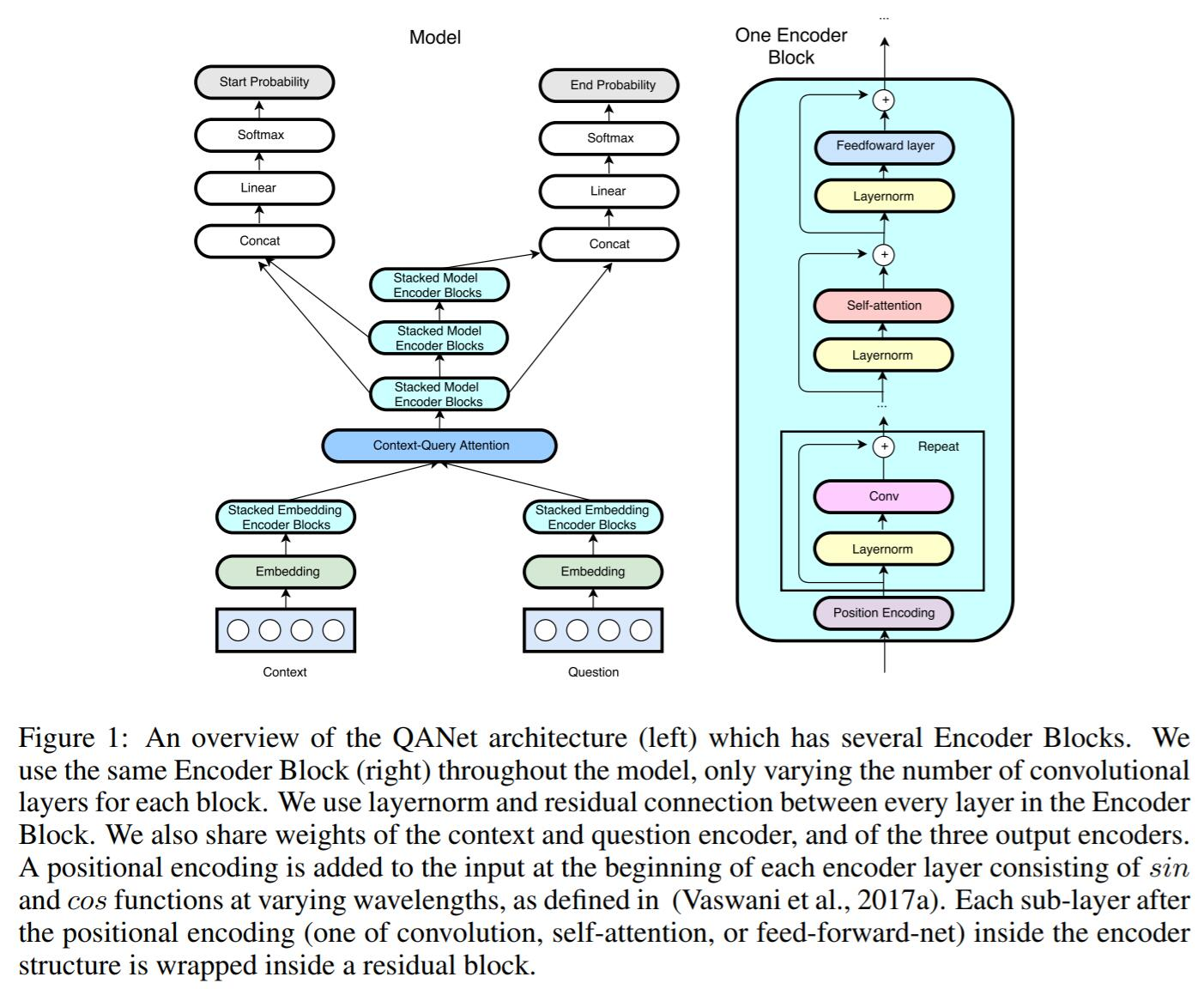图 1 QANet 模型结构

### Input embedding layer

word embedding + character embedding，word embedding 从预先训练好的词向量中读取，每个词向量维度为 $p_1$ ，假设词 $w$ 对应的词向量为 $x_w$；

character embedding 随机初始化，维度为 $p_2$，将每个词的长度 padding or truncating 到 $k$，则词 $w$ 也可以表示成一个 $p_2 * k$ 的矩阵，经过卷积和 max-pooling 后得到一个 $p_2$ 维的 character-level 的词向量，记为 $x_c$。

将 $x_w$ 和 $x_c$ 拼接，得到词 $w$ 对应词向量 $[x_w;x_c]\in{R^{p_1+p_2}}$，最后将拼接的词向量通过一个两层的 highway network，其输出即为 embedding 层的输出。训练过程中，word embedding 固定，character embedding 随模型一起训练。

这里简单介绍一下 highway network ，它主要用于解决深层网络难以训练的问题。

普通的单一网络层可以定义为：

$y=H(x, W_H)$

其中 $H$ 为非线性变换函数，$x$ 为输入，$W_H$ 为网络层权重。

而 highway network 定义如下：

$y=H(x, W_H) * T(x, W_T) + x * C(x, W_C)$

其中 $T$ 称为 transform gate，$C$ 称为 carry gate。直观上来看就是网络层输出一部分来源于非线性变换 $H$ 的输出，另一部分则直接来源于网络层输入 $x$。一般情况下直接令 $C=1-T$，即：

$y=H(x, W_H) * T(x, W_T) + x * (1-T(x, W_T))$

此时显然有：

$y=\begin{cases} x &\text{if T(x, W_T)=0} \\ H(x, W_H) &\text{if T(x, W_T)=1} \end{cases}$

### Embedding Encoder Layer

由 encoder block 组成（这部分只用到了 1 个 encoder block），单个 encoder block 结构自底向上依次包含位置编码 (position encoding)，卷积 (conv) 层，self attention 层和前馈网络 (fnn) 层，具体参考图 1 右侧 one encoder block。每个基本操作 (conv/self-attention/ffn) 都放在残差块 (residual block) 里，具体各层参数参考原论文。原论文对这里卷积层和 self-attention 层的作用解释是：卷积能够捕获上下文局部结构，而 self-attention 则可以捕捉文本之间全局的相互作用。

下面介绍一下各层用到的一些来源于其他论文的概念。

position encoding

直接通过公式计算位置向量，见 Google 的 Attention is All You Need 相关部分。

depthwise separable convolutions

相比普通卷积它所需的参数更少，泛化能力更强。它的核心思想是将一个完整的卷积运算分解为 depthwise convolution 和 pointwise convolution 两个步骤。

首先是 depthwise convolution，以图 2 为例，假设输入是 64 * 64 的 3 通道图片，使用 3 * 3 * 1 大小的卷积核，filter 数量等于上一层的 depth，对输入的每个 channel 独立进行卷积运算，得到与上层 depth 相同个数的 feature map。对应到图里即 3 个 filter 分别对 3 个 channel 进行卷积，得到 3 个 feature map。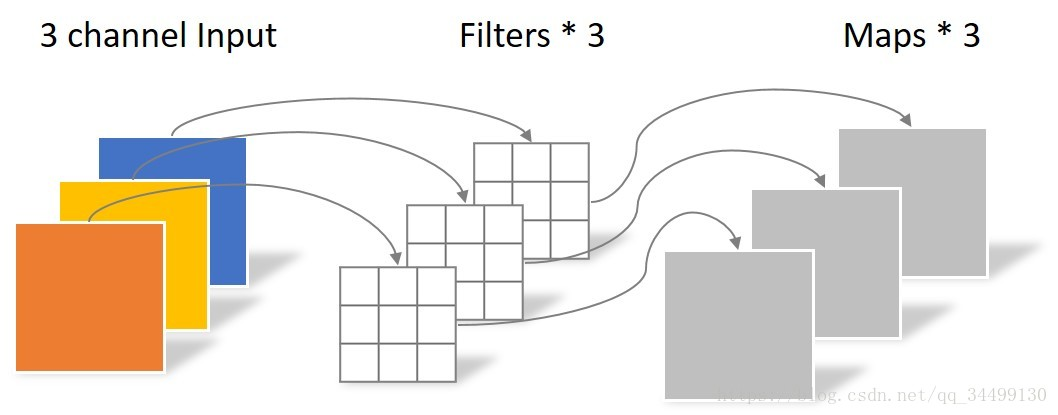图 2 depthwise convolution

然后是 pointwise convolution，和常规卷积运算类似，卷积核的尺寸为 1 * 1 * M，M 等于上一层的 depth。它会将上一步的 feature map 在深度方向上进行加权组合，生成新的 feature map，有几个 filter 就有几个 feature map。如图 3 所示，输入 depth 为 3，卷积核大小为 1 * 1 * 3，使用 4 个 filter，最终生成 4 个 feature map。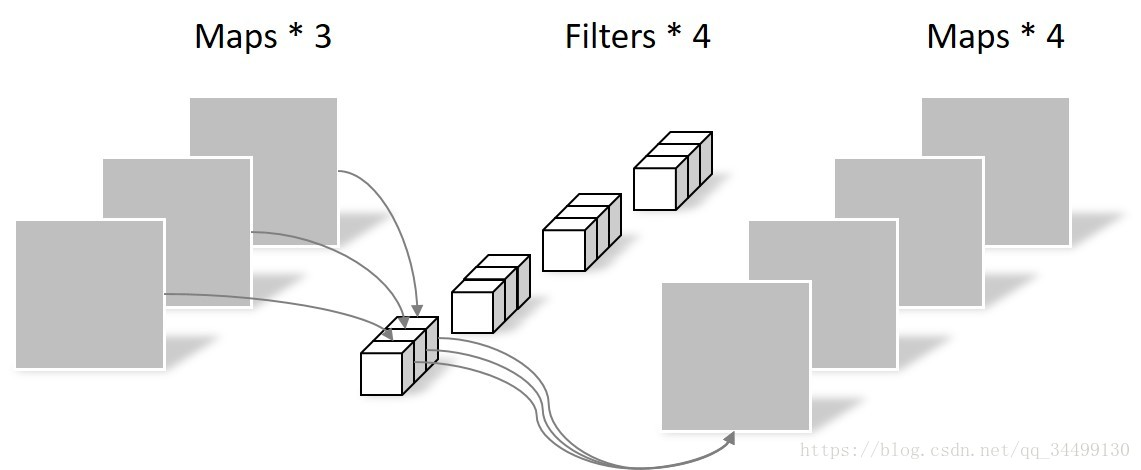图 3 pointwise convolution

直观动画见 这里

self-attention

先简单介绍下 Attention 机制。Attention 机制的基本原理如图 4 所示，对于给定的 Query，计算 Query 和各个 Key 之间的相似度（常用的相似度函数有点积，拼接，感知机等），得到每个 Key 对应的 Value 的权重，用 softmax 函数对权重进行归一化，最后将权重和对应的 Value 进行加权求和得到 Attention 数值。用公式表示就是：

$Attention(Q, K, V)=softmax(Similarity(Q, K)) * V$

对应到 NLP 任务里，可以将句子中的每一个单词看作一个 (Key, Value) 对，则原句子可表示为一系列 (Key,Value) 对。分别计算 Query 和每一个 Key 的相似性，得到每个 Key 对应的 Value 的权重，再对 Value 进行加权求和，得到 Attention 值。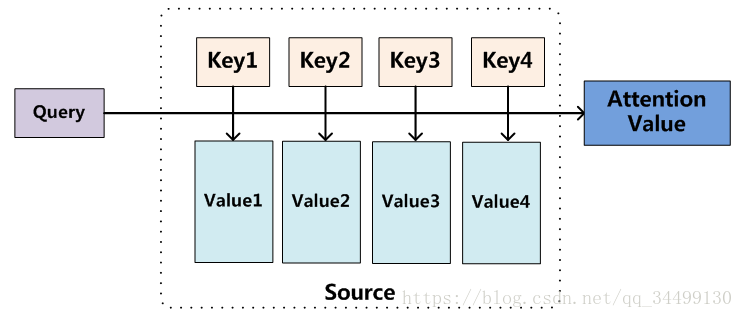图 4 Attention 机制

目前在 NLP 任务中，Key 和 Value 一般都是同一个，即 Key=Value。而 self-attention 则更进一步，令 Q=K=V，使得对于输入的句子，它的任意两个单词之间都要计算 Attention，所以两个单词之间无论有多长距离，最大的路径长度也都只是 1 ，因此 self-attention 可以有效捕获长距离依赖关系，如图 5（来源于原论文附录部分）所示。另外 self-attention 不像 RNN 一样依赖于前一时刻的状态，因此它有利于并行计算。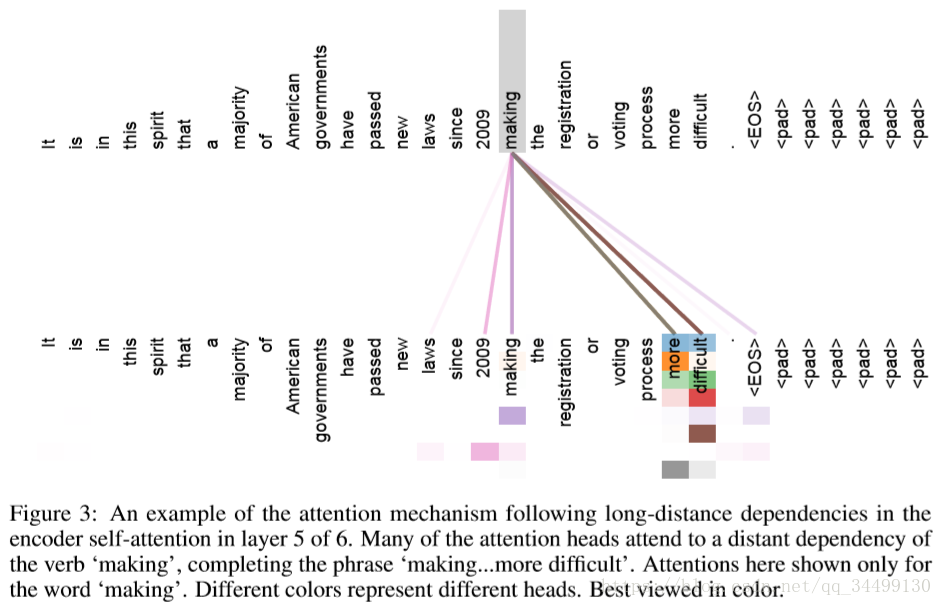图 5 可视化 self-attention

将 Q、K、V 通过参数矩阵映射为多个不同的 Q、K、V 并分别计算 Attention，最后将结果拼接到一起，这里借用 Attention is All You Need 里的表示方法：

$head_i=Attention(Q{W_i}^Q, K{W_i}^K, V{W_i}^V)\\ MultiHead(Q, K, V)=Concat(head_1, ..., head_h)$

其中 ${W_i}^Q\in R^{d_{model} * d_k}$，${W_i}^K\in R^{d_{model} * d_k}$，${W_i}^V \in R^{d_{model} * d_v}$，表示将 $d_{model}$ 维的 Q、K、V 分别映射到 $d_k$、$d_k$、$d_v$ 维。

通过降维可以使 h 个 Attention 的计算量总和与原来 1 个 Attenion 计算量相当，但 h 个 Attention 可以并行，因此大大加快了计算速度。至于 mutil-head attention 的作用，论文原话是

Multi-head attention allows the model to jointly attend to information from different representation subspaces at different positions. With a single attention head, averaging inhibits this.

residual block

出自微软的 Deep Residual Learning for Image Recognition，主要用于缓解深层神经网络梯度消失/爆炸问题和 degradation 现象（具体见原论文）。

residual block 结构如图 6。假定神经网络层的输入是 $x$，期望输出是 $H(x)$，显然 $H(x)$ 学习难度是比较大的。但如果直接把输入 $x$ 传到输出作为初始结果，那么此时需要学习的目标就变成了 $F(x)=H(x)-x$，相当于将优化目标从完整输出替换为为最优解 $H(x)$ 和全等映射 $x$ 的差值，有效降低了学习难度。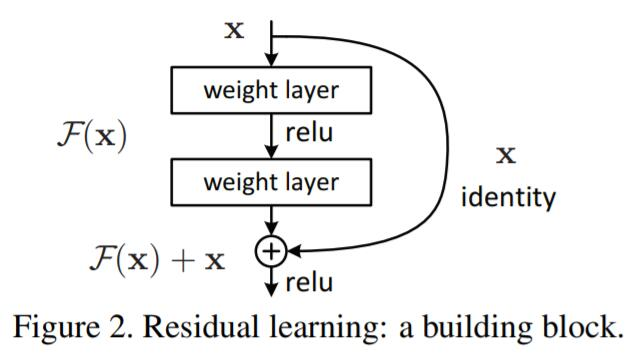图 6 residual block 结构

另外在本论文中，由于 encoder block 中每层之间还用到了 layer normalization，因此对于一个给定的输入 $x$ 和一个操作 $f$，其输出为 $f(layernorm(x))+x$。

layer normalization

### Context-Query Attention Layer

根据上一层得到的 context 和 query 的 encoder 表示来计算 context-to-query attention 和 query-to-context attention 矩阵。分别用 $C$ 和 $Q$ 来表示编码后的 context 和 query，$C\in{R^{d * n}}$、$Q\in{R^{d * m}}$。

1. 首先计算 context 和 query 单词之间的相似度，结果矩阵记为 $S$，$S\in{R^{n * m}}$。其中相似度计算公式为：
$f(q,c)=W_0[q,c,q⊙c]$

$q$、$c$ 分别为单个单词的中间表示，$W_0$ 是一个可训练的参数。

1. 用 softmax 对 $S$ 的行、列分别做归一化得到 $\bar{S}$、$\bar{\bar{S}}$，则 context-to-query attention 矩阵 $A=\bar{S}·Q^T \in {R^{n * d}}$，query-to-context attention 矩阵 $B=\bar{S}·\bar{\bar{S}}^T·C^T \in {R^{n * d}}$（参考 DCN 模型）。

### Model Encoder Layer

由3个 model encoder block 组成，每个 model encoder block 由 7 个 encoder block（见 3.2 节）堆叠而成，3 个 model encoder block 之间共享参数。与 BIDAF 类似，每个位置的输入是 $[c,a,c⊙a,c⊙b]$，$a$ 和 $b$ 分别为 attention 矩阵 $A$ 和 $B$ 的行，$c$ 是 context 对应位置的单词中间表示。

### Output layer

分别预测每个位置是 answer span 的起始点和结束点的概率，分别记为 $p^1$、$p^2$，计算公式如下：

$p^1=softmax(W_1[M_0;M_1]) \\ p^2=softmax(W_2[M_0;M_2])$

其中 $W_1$、$W_2$ 是两个可训练变量，$M_0$、$M_1$、$M_2$ 依次对应结构图中三个 model encoder block 的输出（自底向上）。

目标函数：

$L(θ)=-\frac{1}{N}{\sum_i^N[log(p_{y_i^1}^1)+log(p_{y_i^2}^2)]}$

其中 $y_i^1$、$y_i^2$ 分别为第 $i$ 个样本的 groudtruth 的起始位置和结束位置。

## Reference

 Seo, Minjoon, et al. “Bidirectional attention flow for machine comprehension.” arXiv preprint arXiv:1611.01603 (2016).

 Srivastava, R. K., Greff, K., & Schmidhuber, J. (2015). Highway networks. arXiv preprint arXiv:1505.00387.

 Vaswani, Ashish, et al. “Attention is all you need.” Advances in Neural Information Processing Systems. 2017.

 He, Kaiming, et al. “Deep residual learning for image recognition.” Proceedings of the IEEE conference on computer vision and pattern recognition. 2016.

 深度学习中的注意力机制

## 附：

Tensorflow实现：https://github.com/NLPLearn/QANet

Pytorch实现：https://github.com/hengruo/QANet-pytorch